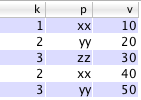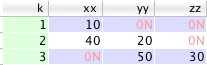# Pivot tables¶

Some notes on the theory and practice of pivoting tables.

## Simple pivot example¶

Given a source table

q)t:([]k:1 2 3 2 3;p:xxyyzzxxyy;v:10 20 30 40 50)we want to obtain

q)pvt:([k:1 2 3]xx:10 40 0N;yy:0N 20 50;zz:0N 0N 30)As originally suggested by Jeff Borror, we begin by getting the distinct pivot values – these will become our column names in addition to the key column k. Note that p must be a column of symbols for this to work.

q)P:asc exec distinct p from t;

And then create the pivot table!

q)pvt:exec P#(p!v) by k:k from t;

which can be read as: for each key k, create a dictionary of the present columns p and their values v, take the full list of columns from that dict, and finally collapse the list of dicts to a table.

Another variation on creating the pivot table

q)pvt:exec P!(p!v)P by k:k from t;

## Explanation¶

A key point to remember is that a table is a list of dictionaries and that is key to how we build the resulting pivot table. A list of conforming dictionaries (same symbol keys, value types) collapses to a table.

q)pvt:((kxxyyzz!1 10 0N 0N);(kxxyyzz!2 40 20 0N);(kxxyyzz!3 0N 50 30))

It’s helpful to play around with these constructs at the q prompt.

q)exec p!v from t
xxyyzzxxyy!10 20 30 40 50

Extract key/value pairs for p and v grouped by k

q)exec p!v by k from t
1 2 3!(enlist xx!enlist 10;yyxx!20 40;zzyy!30 50)

Create a list of dictionaries

q)exec p!v by k:k from t
(flip (enlist k)!enlist 1 2 3)!(enlist xx!enlist 10;yyxx!20 40;zzyy!30 50)

In the dictionaries create nulls for missing values to allow them to conform with common column names and collapse to a table

q)exec P#(p!v) by k:k from t
(+(,k)!,1 2 3)!+s#xxyyzz!(10 40 0N;0N 20 50;0N 0N 30)

## A very general pivot function, and an example¶

Credit

The following is derived from a thread on the k4 listbox between Aaron Davies, Attila Vrabecz and Andrey Zholos.

Create sample data set of level-2 data at 4 quotes a minute, two sides, five levels, NSYE day

q)qpd:5*2*4*"i"$16:00-09:30 q)date:raze(100*qpd)#'2009.01.05+til 5 q)sym:(raze/)5#enlist qpd#'100?4 q)sym:(neg count sym)?sym q)time:"t"$raze 500#enlist 09:30:00+15*til qpd
q)time+:(count time)?1000
q)side:raze 500#enlist raze(qpd div 2)#enlist"BA"
q)level:raze 500#enlist raze(qpd div 5)#enlist 0 1 2 3 4
q)level:(neg count level)?level
q)price:(500*qpd)?100f
q)size:(500*qpd)?100
q)quote:([]date;sym;time;side;level;price;size)

/ pivot t, keyed by k, on p, exposing v
/ f, a function of v and pivot values, names the columns
/ g, a function of k, pivot values, and the return of f, orders the columns
/ either can be defaulted with (::)
/ conceptually, this is
/ exec f$v;P$!raze((flip(p0;p1;.))!/:(v0;v1;..))$;P$by k0,k1,.. from t
/ where P~exec distinct flip(p0;p1;..)from t
/ followed by reordering the columns and rekeying

piv:{[t;k;p;v;f;g]
v:(),v;
G:group flip k!(t:.Q.v t)k;
F:group flip p!t p;
count[k]!g[k;P;C]xcols 0!key[G]!flip(C:f[v]P:flip value flip key F)!raze
{[i;j;k;x;y]
a:count[x]#x 0N;
a[y]:x y;
b:count[x]#0b;
b[y]:1b;
c:a i;
c[k]:first'[a[j]@'where'[b j]];
c}[I[;0];I J;J:where 1<>count'[I:value G]]/:\:[t v;value F]}

q)f:{[v;P]\$raze each string raze P[;0],'/:v,/:\:P[;1]}
q)g:{[k;P;c]k,(raze/)flip flip each 5 cut'10 cut raze reverse 10 cut asc c}
/ BpriceiBsizeiApriceiAsizei for levels i

Use a small subset for testing

q)q:select from quote where sym=first sym
q)book:piv[q;datesymtime;sidelevel;pricesize;f;g]
q)![book;();datesym!datesym;{x!fills,'x}cols get book];
q)book`

One user reports:

This is able to pivot a whole day of real quote data, about 25 million quotes over about 4000 syms and an average of 5 levels per sym, in a little over four minutes.